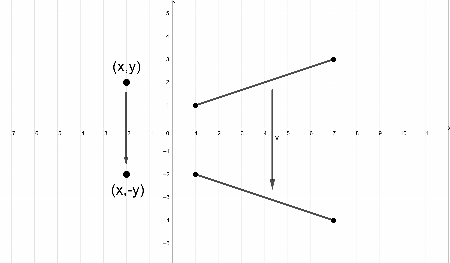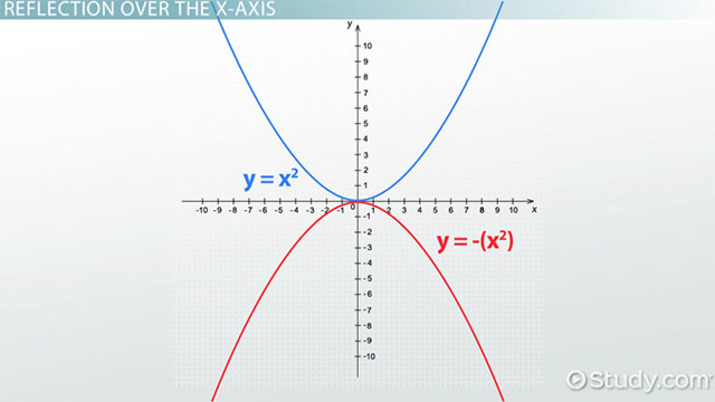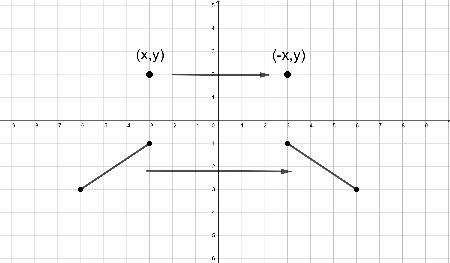Math Courses / Course / Chapter

# Reflection Over the X-Axis and Y-Axis

Riley Kench, Artem Cheprasov
• Author
Riley Kench

Riley has tutored collegiate mathematics for seven years. They have a Master of Arts degree in Mathematics from Central Michigan University and a Bachelor of Science degree in Mathematics from Central Michigan University.

• Instructor
Artem Cheprasov

Artem has a doctor of veterinary medicine degree.

Learn how to reflect over the x-axis and y-axis. See how an equation would be reflected over the y-axis and x-axis with graph examples. Updated: 01/25/2022

Show

## How Do You Reflect Over the X-Axis?

What is a reflection over the x-axis? Firstly, a reflection is a type of transformation representing the flip of a point, line, or curve. The different figures in mathematics can be reflected about points, lines, or even planes, but for this lesson, the only reflections considered will be about lines.

A reflection over the x-axis is a reflection in which the line of reflection is the x-axis. The figure in question is reflected over the x-axis. That is, the points of the figure are reflected over the x-axis and no other line.

How do you reflect over the x-axis? The formula for reflecting a point or line segment is as follows:

• A point {eq}(x,y) {/eq} being reflected over the x-axis will be reflected to the point {eq}(x,-y) {/eq}. That is, the x-value of the coordinate is unchanged and the y-value of the coordinate changes signs. This formula will work for points, line segments, and other figures that arise. Here is an image in Figure 1 showing a point and a line being reflected over the x-axis:In the image in Figure 1, the x-coordinates of the point are fixed throughout the reflection, and the y-coordinate changes signs. This formula will work for any number of points, as shown by the reflection of the line segment. The reflection of the segment requires the same process, but it is applied to every point on the segment. Therefore, it is possible to reflect any figure (line, curve, etc.) across the x-axis using this simple formula.

Finally, does a reflection across the x-axis make intuitive sense? Any point being reflected across the x-axis will only change the y-coordinate since the reflection is only a vertical transformation. Therefore, the x-coordinate should remain unchanged. The distance the point is from the x-axis is the y-value of the coordinate, so reflecting the point should just change the sign of the y-coordinate.

### Reflection over X-Axis for an Equation

Given an equation or function, how does one reflect it over the x-axis? Since the x-coordinate of a point on the curve will remain unchanged through the reflection, but the y-coordinate of a point on the curve will change sign, simply change the sign of the y-variable in the equation. This will produce a curve that, when graphed, is a reflection of the original curve across the x-axis.

Here are the general rules for the reflection over x-axis equation:

• Given an equation, {eq}y=f(x) {/eq}, the reflection equation of the new reflected graph will be {eq}y=-f(x) {/eq}. That is, the function is simply multiplied by {eq}-1 {/eq} which will produce a curve reflected over the x-axis. This is the new reflection over x-axis equation.

The outputs of the equation are multiplied by -1, since only the y-coordinate is changed and it represents the outputs.

Here are the general rules for the reflection over the x-axis of a linear equation and a quadratic equation:

• Given a linear equation {eq}y=mx+b {/eq}, the reflection equation will be {eq}y=-mx-b {/eq} since {eq}-1(mx+b) = -mx-b {/eq}.
• Given a quadratic equation {eq}y=ax^2+bx+c {/eq}, the reflection equation will be {eq}y=-ax^2-bx-c {/eq} since {eq}-1(ax^2+bx+c) = -ax^2-bx-c {/eq}.

Ultimately, in order to reflect an equation over the x-axis, multiply the equation by {eq}-1 {/eq}: {eq}y=f(x) \rightarrow y=-f(x) {/eq}.

Here are some examples of how to reflect different equations across the x-axis:

• If {eq}y=2x-1 {/eq} is reflected over the x-axis, then its new reflection equation is {eq}y=-2x+1 {/eq}.
• If {eq}f(x) = 2x^2-3x+5 {/eq} is reflected over the x-axis, then its new reflection equation is {eq}f(x) = -2x^2+3x-5 {/eq}.An error occurred trying to load this video.

Try refreshing the page, or contact customer support.

Coming up next: Parabolas in Standard, Intercept, and Vertex Form

### You're on a roll. Keep up the good work!

Replay
Your next lesson will play in 10 seconds
• 0:01 Reflections &…
• 0:39 Reflection Over the X-Axis
• 1:49 Reflection Over the Y-Axis
• 3:00 Lesson Summary
Save Save

Want to watch this again later?

Timeline
Autoplay
Autoplay
Speed Speed

## How To Reflect Over The Y-Axis

Reflection over the y-axis is very similar to reflection over the x-axis. In this case, the reflection is performed about the y-axis. So, the points of the figure being reflected are reflected over the y-axis and no other lines.

Here is the general formula for reflecting across the y-axis:

• A point {eq}(x,y) {/eq} being reflected over the y-axis will be reflected to {eq}(-x,y) {/eq}.

The y-coordinate in this case is unchanged, but the x-coordinate changes sign. The intuition behind this reflection is similar to the case of the x-axis reflection since the distance to the y-axis is represented by the x-coordinate and that is the only coordinate to be changed.

Here is an image in Figure 2 showing a point and a line segment being reflected across the y-axis:In the image in Figure 2, only the x-coordinate is changed, while the y-coordinate remains unchanged. This is because the reflection is purely horizontal, so it will only affect the x-coordinate.

### Reflection over Y-Axis for an Equation

How to reflect over y-axis given an equation or function? In the case of the x-axis reflection, the equation is simply multiplied by {eq}-1 {/eq} in order to produce the new reflection equation. This is because the y-variable is the one being transformed. In this case, the x-variable is the one being transformed. Therefore, the x-variable must be manipulated in order to produce the reflection over y-axis equation.

Here is the general rule for reflection across the y-axis:

• Given an equation {eq}y=f(x) {/eq}, the new reflection equation of the reflected graph will be {eq}y=f(-x) {/eq}.

The inputs of the equation are being multiplied by {eq}-1 {/eq} since the x-coordinates represent the inputs of the equation.

Here are the general rules for reflecting linear equations and quadratic equations over the y-axis:

• Given a linear equation {eq}y=mx+b {/eq}, the reflection equation will be {eq}y=-mx+b {/eq} since {eq}m(-x)+b = -mx+b {/eq}.
• Given a quadratic equation {eq}y=ax^2+bx+c {/eq}, the reflection equation will be {eq}y=ax^2-bx+c {/eq} since {eq}a(-x)^2+b(-x)+c = ax^2-bx+c {/eq}.

Ultimately, when reflecting over the y-axis, replace the x-variable in the equation with {eq}-x {/eq}. This will produce a new equation that is reflected over the y-axis.

Here are some examples of different equations being reflected across the y-axis:

• If {eq}y=5x-5 {/eq} is reflected over the x-axis, then its new reflection equation will be {eq}y = 5(-x)-5 = -5x-5 {/eq}.
• If {eq}f(x) = -2x^2+3x-7 {/eq} is reflected across the y-axis, then its new reflection equation will be {eq}f(x) = -2(-x)^2+3(-x)-7 = -2x^2-3x-7 {/eq}.

## Reflection over Y=X and Y=-X

Two more commonly used lines of reflection that arise in mathematics are the lines {eq}y=x {/eq} and the line {eq}y=-x {/eq}. These are the main diagonals of the plane. Here is how to reflect over the line {eq}y=x {/eq}:

• To reflect over the line {eq}y=x {/eq} if given a point {eq}(x,y) {/eq}, simply interchange the coordinates: {eq}(x,y) \rightarrow (y,x) {/eq}.

The above formula works for reflecting points, but the process for reflecting an equation is more difficult:

To unlock this lesson you must be a Study.com Member.

#### What is the formula for reflection over Y =- X?

The formula for reflecting over the line y=-x first involves switching the variables: (x,y) becomes (y,x). Then, one must change the signs of each of the variables: (y,x) then becomes (-y,-x). For an equation, first switch the variables in the equation so that x becomes y and y becomes x. Then solve for the dependent variable y. Finally, multiply the input and output variables (x and y) by -1: y=f(x) becomes y=-f(-x).

#### What is the formula for reflection over the x-axis?

The formula for reflection over the x-axis is to change the sign of the y-variable of the coordinate point. The point (x,y) is sent to (x,-y). For an equation, the output variable is multiplied by -1: y=f(x) becomes y=-f(x).

### Register to view this lesson

Are you a student or a teacher?

Back

### Resources created by teachers for teachers

Over 30,000 video lessons & teaching resources‐all in one place.Video lessonsQuizzes & WorksheetsClassroom IntegrationLesson Plans

I would definitely recommend Study.com to my colleagues. It’s like a teacher waved a magic wand and did the work for me. I feel like it’s a lifeline.

Jennifer B.
TeacherCreate an account to start this course today
Used by over 30 million students worldwide